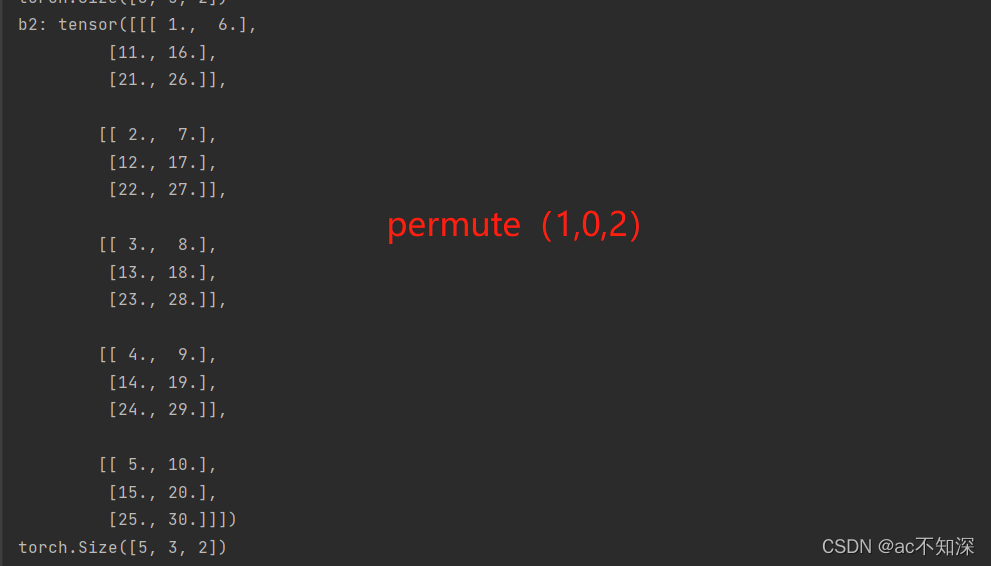﻿ pytorch中permute()函数用法补充说明(矩阵维度变化过程)_python_脚本之家
python# pytorch中permute()函数用法补充说明(矩阵维度变化过程)

## 二、举例解释

————————————————分割线——————————————

```b = x.permute(2,1,0)            # 交换块和列
print(b)
print(b.size())
```————————————————分割线——————————————

```维度数值变换：(3,2,5)==>(3,5,2)==>(5,3,2)==>(5,2,3)
# 读者一定要考虑清楚下一行代表什么意思

# 第一个"==>" 变化为2和5交换，对应函数里的参数0不动，1和2交换 ，变换完后为(0,2,1)，对应维度是(3,5,2)。重排序为(0,1,2)
# 第二个"==>" 变化为3和5交换，对应函数里的参数2不动，0和1交换，变换完后为(1,0,2)，对应维度是(5,3,2)。重排序为(0,1,2)
# 第三个"==>" 变化为3和2交换，对应函数里的参数0不动，1和2交换，变换完后为(0,2,1)，对应维度是(5,2,3)。重排序为(0,1,2)
```

(3,2,5)-(0,1,2)
(3,5,2)2和5(0,1,2)==> (0,2,1)
(5,3,2)3和5(0,1,2)==>(1,0,2)
(5,2,3)3和2(0,1,2)==>(0,2,1)

```import torch

x = torch.linspace(1,30,steps=30).view(3,2,5)
print('x:',x)
print(x.size())

# permute(0,1,2)==>permute(2,1,0)
# (3,2,5)==>(3,5,2)==>(5,3,2)==>(5,2,3)
#
# (0,1,2)==>(0,2,1)==>(1,0,2)==>(0,2,1)

b1 = x.permute(0,2,1)
print('b1:',b1)
print(b1.size())

b2 = b1.permute(2,0,1)
print('b2:',b2)
print(b2.size())

b = b2.permute(2,1,0)
print('b:',b)
print(b1.size())```

### 1、permute(0,1,2)### 2、permute(0,1,2) ⇒ permute(0,2,1)2行3列==>3行2列

### 3、permute(0,2,1) ⇒ permute(1,0,2)### 4、permute(1,0,2) ⇒ permute(0,2,1)## 三、写在最后